CBSE Class 10 Sample Paper for 2022 Boards - Maths Standard [Term 2]

Class 10
Solutions of Sample Papers for Class 10 Boards

## From a point P, two tangents PA and PB are drawn to a circle C (0, r). ∠If OP = 2r, then find ∠APB. What type of triangle is APB?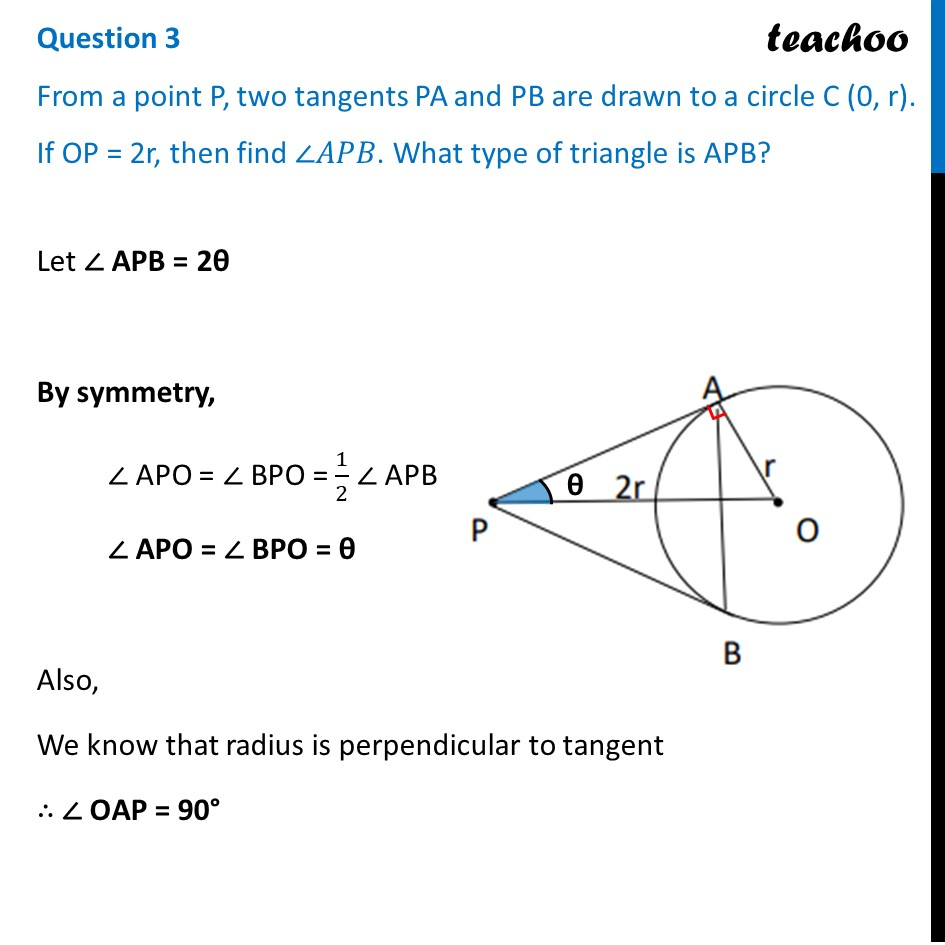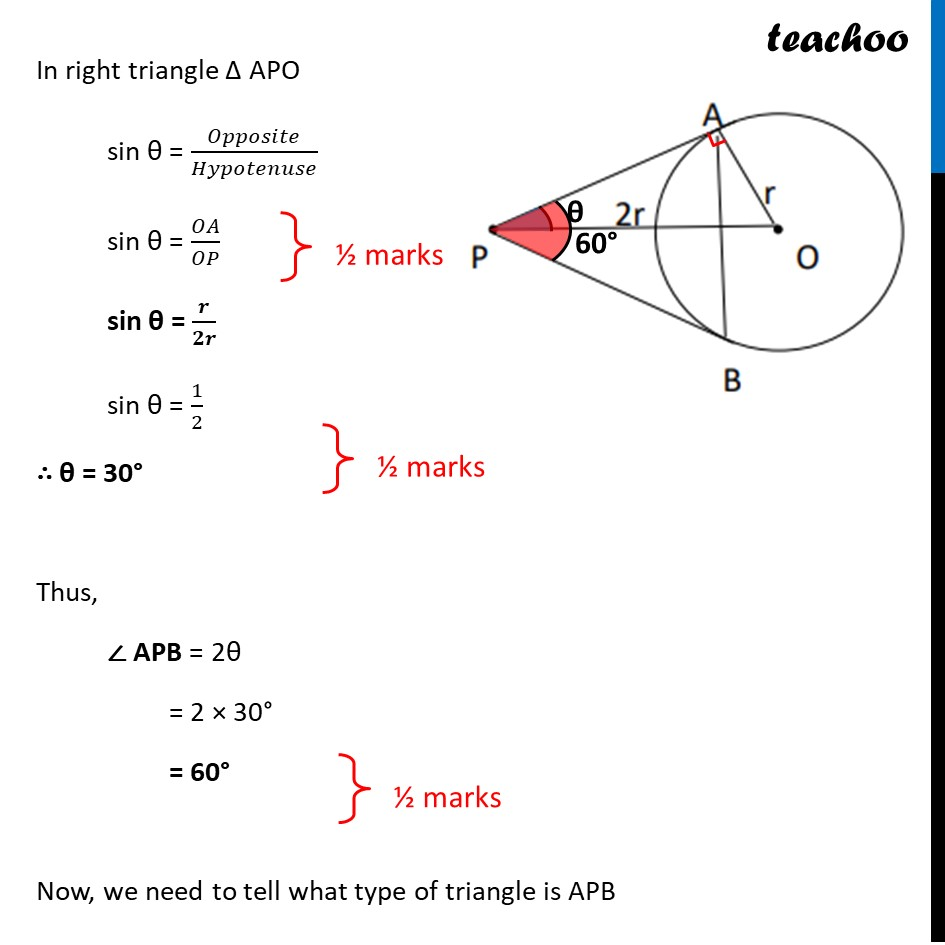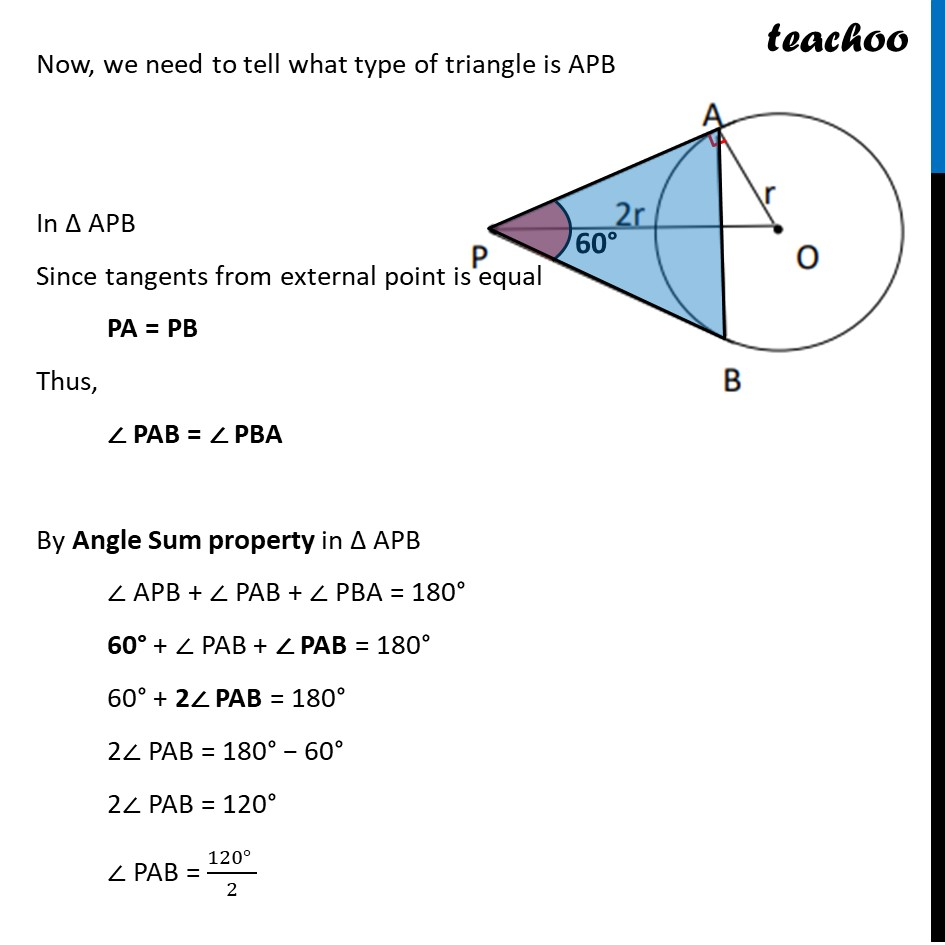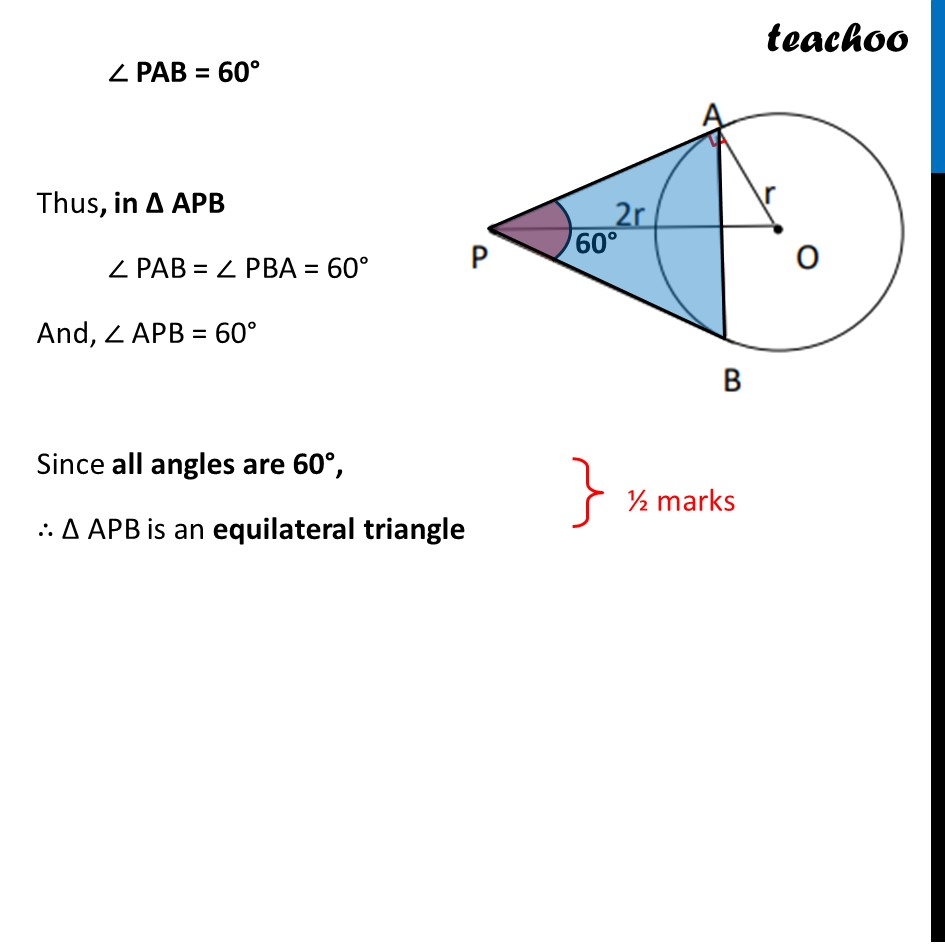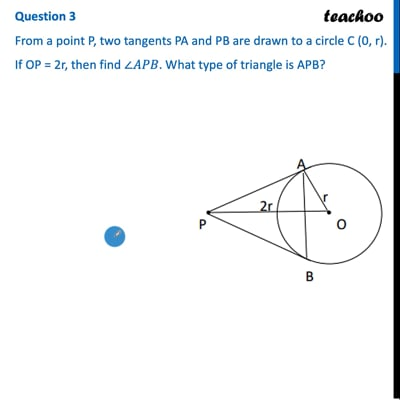This video is only available for Teachoo black users

### Transcript

Question 3 From a point P, two tangents PA and PB are drawn to a circle C (0, r). If OP = 2r, then find ∠𝐴𝑃𝐵. What type of triangle is APB? Let ∠ APB = 2θ By symmetry, ∠ APO = ∠ BPO = 1/2 ∠ APB ∠ APO = ∠ BPO = θ Also, We know that radius is perpendicular to tangent ∴ ∠ OAP = 90° In right triangle Δ APO sin θ = 𝑂𝑝𝑝𝑜𝑠𝑖𝑡𝑒/𝐻𝑦𝑝𝑜𝑡𝑒𝑛𝑢𝑠𝑒 sin θ = 𝑂𝐴/𝑂𝑃 sin θ = 𝒓/𝟐𝒓 sin θ = 1/2 ∴ θ = 30° Thus, ∠ APB = 2θ = 2 × 30° = 60° Now, we need to tell what type of triangle is APB Now, we need to tell what type of triangle is APB In Δ APB Since tangents from external point is equal PA = PB Thus, ∠ PAB = ∠ PBA By Angle Sum property in Δ APB ∠ APB + ∠ PAB + ∠ PBA = 180° 60° + ∠ PAB + ∠ PAB = 180° 60° + 2∠ PAB = 180° 2∠ PAB = 180° − 60° 2∠ PAB = 120° ∠ PAB = (120° )/2 ∠ PAB = 60° Thus, in Δ APB ∠ PAB = ∠ PBA = 60° And, ∠ APB = 60° Since all angles are 60°, ∴ Δ APB is an equilateral triangle